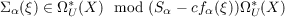#### Vol. 33, No. 1, 1970

 Download this articleFor screen For printingRecent Issues Vol. 325: 1 Vol. 324: 1  2 Vol. 323: 1  2 Vol. 322: 1  2 Vol. 321: 1  2 Vol. 320: 1  2 Vol. 319: 1  2 Vol. 318: 1  2Online Archive Volume: Issue:The Journal Subscriptions Editorial Board Officers Contacts Submission Guidelines Submission Form Policies for Authors ISSN: 1945-5844 (e-only) ISSN: 0030-8730 (print) Special Issues Author Index To Appear Other MSP Journals
On secondary characteristic classes in cobordism theory

### Wei-lung Ting

Vol. 33 (1970), No. 1, 249–253
##### Abstract

This paper introduces into cobordism theory a new notion borrowed from ordinary cohomology theory. Specifically, let ξ be a U(n)-bundle over the CW-complex X. Let E and E0 be the total spaces of the associated bundles whose fibers are respectively the unit disc E2n Cn and the unit sphere S2n1 Cn. The classifying map for ξ gives rise to an element Uξ ΩU2n(E,E0). One defines the Thom isomorphism φ : ΩUq(X) ΩUq+2n(E,E0) by φ(x) = (px)Uξ and Euler class, e(ξ) of ξ, by e(ξ) = p∗−1j(Uξ). For each α = (α12,), let ofα(ξ) ΩU2|α|(X) be the Conner-Floyd Chern class of ξ, and Sα : ΩUq(X,Y ) ΩUq+2|α|(X,Y ) be the operation defined by Novikov. Then one has the relation, Sα(e(ξ)) = cfα(ξ) e(ξ). Now if ξ is a bundle such that e(ξ) = 0, then one can define a secondary characteristic classby using the above relation. The object of this paper is to study some of the properties of such secondary characteristic classes.

Primary: 57.32
##### Milestones